•5星
387KB weixin_44510615 2021-06-23 18:04:15
•5星
216KB m0_52957036 2020-02-28 14:17:44
•5星
11.8MB guoruibin123 2021-05-21 15:45:32
•5星
2.28MB qq_40957277 2021-06-22 18:53:14
• 1.75MB netpbc 2020-08-31 21:30:24
• 198KB weixin_43171119 2018-09-10 17:17:27
•3星
224KB qq_41268867 2018-03-05 21:05:41
•5星
307B qq_34683252 2018-04-18 22:21:56
• 基于Kmeans聚类算法实现图像分割（从原理开始实现） 聚类 算法 opencv c++

一、Kmeans聚类算法基本原理 K-Means算法的思想很简单，对于给定的样本集，按照样本之间的距离大小，将样本集划分为K个簇。让簇内的点尽量紧密的连在一起，而让簇间的距离尽量的大。 以彩色图像为例：基于彩色图像的...

一、Kmeans聚类算法基本原理
K-Means算法的思想很简单，对于给定的样本集，按照样本之间的距离大小，将样本集划分为K个簇。让簇内的点尽量紧密的连在一起，而让簇间的距离尽量的大。
以彩色图像为例：基于彩色图像的RGB三通道为xyz轴建立空间直角坐标系，那么一副图像上的每个像素点与该空间直角坐标系建立了一 一映射（双射）的关系。
从空间直角坐标系中随机取 k 个点，作为 k 个簇的各自的中心。计算所有像素点到k个簇心的距离，并将所有像素点划分至与其距离最小的簇类。自此聚类完成。其中，距离定义为欧氏距离：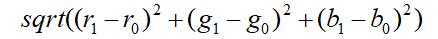其中r，g，b分别表示红绿蓝三通道，r1，g1，b1为彩色图片中某像素点；r0，g0，b0表示某簇类的簇心。
二、基于Kmeans图像分割算法流程
Note：彩色图像的操作是基于一个三维空间
1、加载图像，获取图像的所有像素点并将其转换为样本数据。
2、开始迭代
a)、初始化簇心坐标。
a)、更新簇心坐标，遍历样本数据中的数据点并计算数据点与所有簇心的距离。对于某数据点计算得到的与所有簇类的欧氏距离中，取欧氏距离最小所对应的簇类作为该数据点对应的类。
c)、计算更新后的簇心坐标与更新前的簇心坐标的均方误差。若均方误差仍大于某阈值，则重复b)，反之结束迭代。
Kmeans的详细算法流程参考博文：
https://www.cnblogs.com/pinard/p/6164214.html
三、代码主函数部分
1、加载图片

Mat src, dst;
namedWindow("输入图像", WINDOW_AUTOSIZE);
imshow("输入图像", src);

2、初始化颜色（图像分割后需要上色）

Scalar colorTab[] = {
Scalar(0,0,255),
Scalar(0,255,0),
Scalar(255,0,0),
Scalar(0,255,255),
Scalar(255,0,255),
};

3、初始化簇类数，并将所有的像素点全部转换为样本数据

int sampleCount = width*height;
int clusterCount = 5;
Mat points(sampleCount, dims, CV_32F, Scalar(10));
//将彩色图像的像素点转换为样本数据
int index = 0;
for (int row = 0; row < height; row++)
{
for (int col = 0; col < width; col++)
{
index = row*width + col;
Vec3b bgr = src.at<Vec3b>(row, col);
points.at<float>(index, 0) = static_cast<int>(bgr);//b
points.at<float>(index, 1) = static_cast<int>(bgr);//g
points.at<float>(index, 2) = static_cast<int>(bgr);//r
}
}

4、利用Kmeans算法对样本数据分类

centers, feature = Kmeans(points, clusterCount, sampleCount);

5、
显示图像分割后的结果

//显示图像分割结果
Mat result = Mat::zeros(src.size(), src.type());
int index1 = 0;
for (int row = 0; row < height; row++)
{
for (int col = 0; col < width; col++)
{
index1 = row*width + col;
int label = feature.at<float>(index1, 3);
result.at<Vec3b>(row, col) = colorTab[label];
result.at<Vec3b>(row, col) = colorTab[label];
result.at<Vec3b>(row, col) = colorTab[label];
}
}
imshow("基于Kmeans聚类的图像分割", result);

四、代码子函数部分
1、初始化聚类中心

//初始化簇心
Mat centers = Mat::zeros(clusterCount, 1, CV_32FC3);//4行3列
centers.at<float>(0, 0) = 150;
centers.at<float>(0, 1) = 180;
centers.at<float>(0, 2) = 200;

centers.at<float>(1, 0) = 20;
centers.at<float>(1, 1) = 25;
centers.at<float>(1, 2) = 37;

centers.at<float>(2, 0) = 80;
centers.at<float>(2, 1) = 100;
centers.at<float>(2, 2) = 140;

centers.at<float>(3, 0) = 226;
centers.at<float>(3, 1) = 234;
centers.at<float>(3, 2) = 235;

2、利用Kmeans算法进行迭代

Mat feature = Mat::zeros(sampleCount, 1, CV_32FC4);
float *distance = new float[sampleCount];
int epoch = 0;
while (true)
{
for (int row = 0; row < points.rows; row++)
{
for (int i = 0; i < centers.rows; i++)
{
distance[i] = pow((points.at<float>(row, 0) - centers.at<float>(i, 0)), 2) + pow((points.at<float>(row, 1) - centers.at<float>(i, 1)), 2)
+ pow((points.at<float>(row, 2) - centers.at<float>(i, 2)), 2);
}
float min = distance;
int flag = 0;
for (int i = 0; i < clusterCount; i++)
{
if (min > distance[i])
{
min = distance[i];
flag = i;
}
}
feature.at<float>(row, 0) = points.at<float>(row, 0);
feature.at<float>(row, 1) = points.at<float>(row, 1);
feature.at<float>(row, 2) = points.at<float>(row, 2);
feature.at<float>(row, 3) = flag;
}
float new_center_r = 0, new_center_g = 0, new_center_b = 0;
float *temp = new float[clusterCount];
for (int i = 0; i < clusterCount; i++)
{
int num = 0;
float sum_center_r = 0, sum_center_g = 0, sum_center_b = 0;
for (int row = 0; row < sampleCount; row++)
{
if (feature.at<float>(row, 3) == i)
{
sum_center_b = sum_center_b + feature.at<float>(row, 0);
sum_center_g = sum_center_g + feature.at<float>(row, 1);
sum_center_r = sum_center_r + feature.at<float>(row, 2);
num++;
}
}
new_center_b = sum_center_b / num;
new_center_g = sum_center_g / num;
new_center_r = sum_center_r / num;

temp[i] = pow((new_center_b - centers.at<float>(i, 0)), 2) + pow((new_center_g - centers.at<float>(i, 1)), 2)
+ pow((new_center_r - centers.at<float>(i, 2)), 2);

centers.at<float>(i, 0) = new_center_b;
centers.at<float>(i, 1) = new_center_g;
centers.at<float>(i, 2) = new_center_r;
}
float total = 0;
float mean_square_error = 0;
for (int i = 0; i < clusterCount; i++)
{
total = total + temp[i];
}
mean_square_error = total / 4;
if (epoch % 1 == 0)
cout << "epoch：" << epoch << "\terror of mean square：" << mean_square_error << endl;
if (mean_square_error < 0.01)
break;
epoch++;
}

五、代码运行结果及评价
输入图片：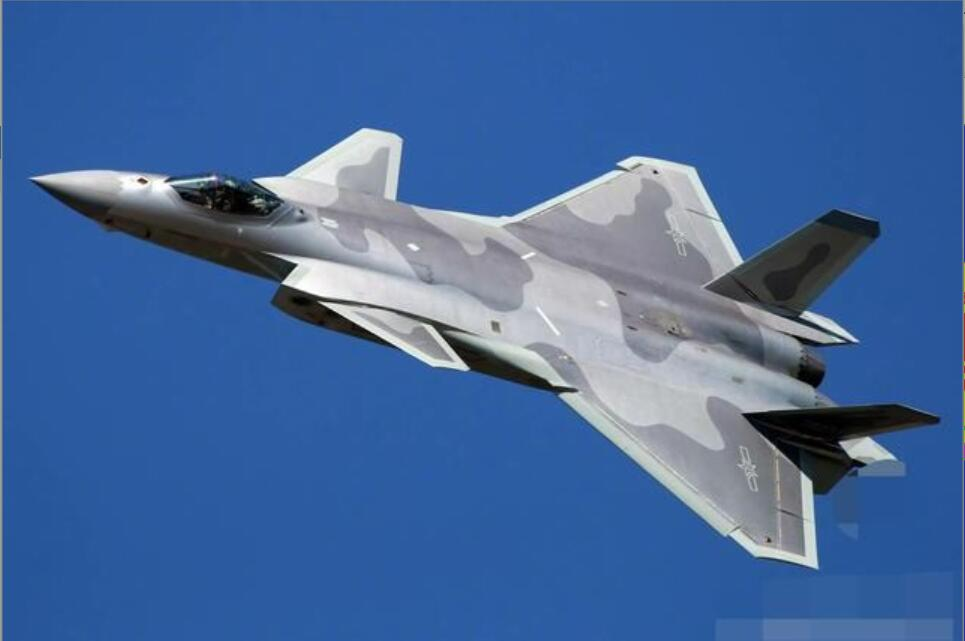2、迭代过程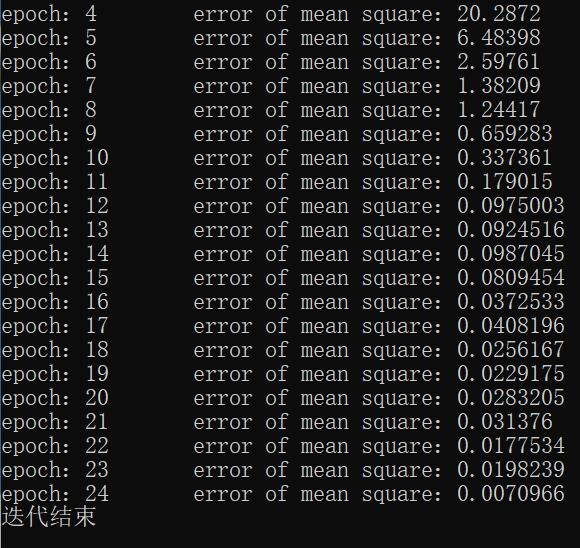3、聚类结果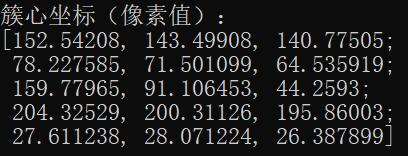如上图，从左至右分别为Blue、Green、Red通道；从上之下分别是五个簇心的坐标（像素值）。
4、图像分割结果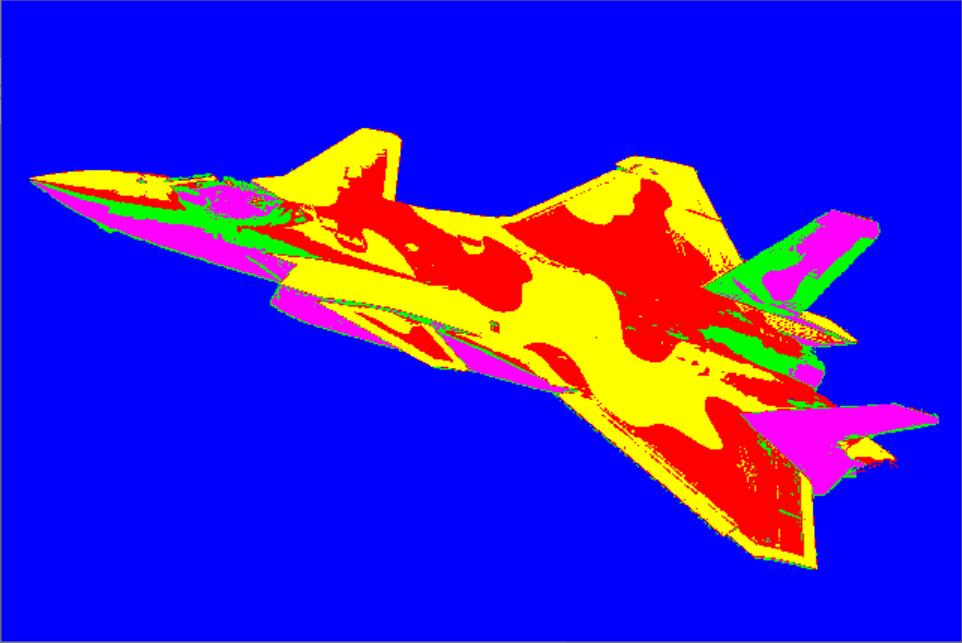如上图，图像被清晰的分为了五个部分：背景为蓝色、歼20的迷彩涂装分为了红黄两色，颜色最暗的地方为紫色，颜色次暗的地方为绿色。
另外，对于大数据，经典的Kmeans算法显然处理速度太慢，但对于彩色图像，使用经典Kmeans算法对其进行分割，其所耗时长在可接受的范围内。
至此完全实现了基于Kmeans聚类算法的图像分割，经验证，其结果与利用OpenCV提供的API得到的效果完全一致！
六、最后附上所有代码

#include<iostream>
#include<opencv2/opencv.hpp>
using namespace std;
using namespace cv;
Mat Kmeans(Mat points, int clusterCount, int sampleCount);
int main()
{
Mat src, dst;
namedWindow("输入图像", WINDOW_AUTOSIZE);
imshow("输入图像", src);

Scalar colorTab[] = {
Scalar(0,0,255),
Scalar(0,255,0),
Scalar(255,0,0),
Scalar(0,255,255),
Scalar(255,0,255),
};
int width = src.cols;
int height = src.rows;
int dims = src.channels();

int sampleCount = width*height;
int clusterCount = 5;
Mat points(sampleCount, dims, CV_32F, Scalar(10));
//将彩色图像的像素点转换为样本数据
int index = 0;
for (int row = 0; row < height; row++)
{
for (int col = 0; col < width; col++)
{
index = row*width + col;
Vec3b bgr = src.at<Vec3b>(row, col);
points.at<float>(index, 0) = static_cast<int>(bgr);//b
points.at<float>(index, 1) = static_cast<int>(bgr);//g
points.at<float>(index, 2) = static_cast<int>(bgr);//r
}
}
Mat feature;
Mat centers;
centers, feature = Kmeans(points, clusterCount, sampleCount);
//显示图像分割结果
Mat result = Mat::zeros(src.size(), src.type());
int index1 = 0;
for (int row = 0; row < height; row++)
{
for (int col = 0; col < width; col++)
{
index1 = row*width + col;
int label = feature.at<float>(index1, 3);
result.at<Vec3b>(row, col) = colorTab[label];
result.at<Vec3b>(row, col) = colorTab[label];
result.at<Vec3b>(row, col) = colorTab[label];
}
}
imshow("基于Kmeans聚类的图像分割", result);
waitKey(0);
system("pause");
return 0;
}
Mat Kmeans(Mat points, int clusterCount, int sampleCount)
{
//初始化簇心
Mat centers = Mat::zeros(clusterCount, 1, CV_32FC3);//4行3列
centers.at<float>(0, 0) = 150;
centers.at<float>(0, 1) = 180;
centers.at<float>(0, 2) = 200;

centers.at<float>(1, 0) = 20;
centers.at<float>(1, 1) = 25;
centers.at<float>(1, 2) = 37;

centers.at<float>(2, 0) = 80;
centers.at<float>(2, 1) = 100;
centers.at<float>(2, 2) = 140;

centers.at<float>(3, 0) = 226;
centers.at<float>(3, 1) = 234;
centers.at<float>(3, 2) = 235;
Mat feature = Mat::zeros(sampleCount, 1, CV_32FC4);
float *distance = new float[sampleCount];
int epoch = 0;
while (true)
{
for (int row = 0; row < points.rows; row++)
{
for (int i = 0; i < centers.rows; i++)
{
distance[i] = pow((points.at<float>(row, 0) - centers.at<float>(i, 0)), 2) + pow((points.at<float>(row, 1) - centers.at<float>(i, 1)), 2)
+ pow((points.at<float>(row, 2) - centers.at<float>(i, 2)), 2);
}
float min = distance;
int flag = 0;
for (int i = 0; i < clusterCount; i++)
{
if (min > distance[i])
{
min = distance[i];
flag = i;
}
}
feature.at<float>(row, 0) = points.at<float>(row, 0);
feature.at<float>(row, 1) = points.at<float>(row, 1);
feature.at<float>(row, 2) = points.at<float>(row, 2);
feature.at<float>(row, 3) = flag;
}
float new_center_r = 0, new_center_g = 0, new_center_b = 0;
float *temp = new float[clusterCount];
for (int i = 0; i < clusterCount; i++)
{
int num = 0;
float sum_center_r = 0, sum_center_g = 0, sum_center_b = 0;
for (int row = 0; row < sampleCount; row++)
{
if (feature.at<float>(row, 3) == i)
{
sum_center_b = sum_center_b + feature.at<float>(row, 0);
sum_center_g = sum_center_g + feature.at<float>(row, 1);
sum_center_r = sum_center_r + feature.at<float>(row, 2);
num++;
}
}
new_center_b = sum_center_b / num;
new_center_g = sum_center_g / num;
new_center_r = sum_center_r / num;

temp[i] = pow((new_center_b - centers.at<float>(i, 0)), 2) + pow((new_center_g - centers.at<float>(i, 1)), 2)
+ pow((new_center_r - centers.at<float>(i, 2)), 2);

centers.at<float>(i, 0) = new_center_b;
centers.at<float>(i, 1) = new_center_g;
centers.at<float>(i, 2) = new_center_r;
}
float total = 0;
float mean_square_error = 0;
for (int i = 0; i < clusterCount; i++)
{
total = total + temp[i];
}
mean_square_error = total / 4;
if (epoch % 1 == 0)
cout << "epoch：" << epoch << "\terror of mean square：" << mean_square_error << endl;
if (mean_square_error < 0.01)
break;
epoch++;
}
delete[] distance;
cout << "迭代结束" << endl;
cout << endl;
cout << "簇心坐标（像素值）：" << endl;
cout << centers << endl;
return centers, feature;
}

展开全文jun_hun_ 2020-03-12 15:53:55
• Kmeans聚类及图像分割 机器学习 聚类 Kmeans 图像分割

Kmeans是最简单的聚类算法之一，应用十分广泛，Kmeans以距离作为相似性的评价指标，其基本思想是按照距离将样本聚成不同的簇，两个点的距离越近，其...本文参考PRML一书，详细讲解Kmeans聚类原理以及图像分割应用。

Kmeans是最简单的聚类算法之一，应用十分广泛，Kmeans以距离作为相似性的评价指标，其基本思想是按照距离将样本聚成不同的簇，两个点的距离越近，其相似度就越大，以得到紧凑且独立的簇作为聚类目标。本文参考PRML一书，详细讲解Kmeans聚类的原理以及图像分割应用。

1. 基本原理

给定 D $D$维欧几里得空间的一组数据{x1,...,xN}$\left\{ {{x_1},...,{x_N}} \right\}$，我们的任务是将该组数据聚成 K $K$个簇（聚类和分类的区别在于分类是有监督的，聚类是无监督的，根据任务设定聚类依据，此处假设聚类个数K是已知的）。不考虑问题背景，单纯从欧几里得空间的角度讲，我们应当将距离较近的点聚为一个簇，不同簇的点之间的距离较远。Kmeans聚类方法就是寻找K$K$个聚类中心 μk(k=1,...,K) $\mu_k\left(k=1,...,K\right)$，将所有的数据分配到距离最近的聚类中心，使得每个点与其相应的聚类中心距离的平方和最小。
我们引入二值变量 rnk{0,1} $r_{nk}\in\left\{0,1\right\}$来表示数据点 xn $x_n$对于聚类 k $k$的归属（其中n=1,...,N$n=1,...,N$, k=1,...,K $k=1,...,K$），如果数据点 xn $x_n$属于第 k $k$聚类，则rnk=1$r_{nk}=1$，否则为 0 $0$。如此，我们便可定义如下损失函数：

J=n=1Nk=1Krnkxnμk2(1)$J=\sum\limits_{n=1}^N{\sum\limits_{k=1}^K}{r_{nk}{\left\|x_n-\mu_k\right\|}^2}\qquad(1)$
该问题的目标就是寻找使得损失函数 J $J$最小的所有数据点的归属值{rnk}$\left\{r_{nk}\right\}$和聚类中心 {μk} $\left\{\boldsymbol{\mu}_k\right\}$。Kmeans算法提供了一种迭代求解方法，在每次迭代中交替优化 rnk $r_{nk}$ μk $\boldsymbol{\mu}_k$
第一步，随机选择聚类中心 μk $\boldsymbol{\mu}_k$的初始值，求取使损失函数 J $J$最小的数据点的归属值rnk$r_{nk}$。由 (1) $\left(1\right)$式容易看出，给定 xn $x_n$ μk $\boldsymbol{\mu}_k$的值，损失函数 J $J$rnk$r_{nk}$的线性函数，而且，由于 xn $x_n$之间是相互独立的，所以对于每一个 n $n$，我们只需将该点分配到距离最近的聚类中心，即
rnk={10if k=argminjxnμj2otherwise(2)
第二步，固定已求得的 rnk $r_{nk}$，再求取使损失函数 J $J$最小的聚类中心μk$\boldsymbol{\mu}_k$。给定 rnk $r_{nk}$的值，损失函数 J $J$μk$\boldsymbol{\mu}_k$的二次函数，令 J $J$μk$\boldsymbol{\mu}_k$的导数为 0 $0$，我们有
n=1Nrnk(xnμk)=0(3)$\sum\limits_{n=1}^N{r_{nk}\left(x_n-\boldsymbol{\mu}_k\right)}=0\quad(3)$
那么 μk $\boldsymbol{\mu}_k$的取值为

μk=nrnkxnnrnk(4)
对于第 k $k$个聚类，rnk$r_{nk}$取1的个数就是属于该聚类的点的个数，因此， μk $\boldsymbol{\mu}_k$等于属于该聚类的点均值。
如此迭代该两阶段优化问题直至收敛,Kmeans的实现过程大致表示如下：
(1) 随机选取K个初始聚类中心；
(2) 计算每个样本到各聚类中心的距离，将每个样本归到其距离最近的聚类中心；
(3) 对每个簇，以所有样本的均值作为该簇新的聚类中心；
(4) 重复第(2)~(3)步,直到聚类中心不再变化或达到设定的迭代次数。
图1为Kmeans算法的实现过程图示，以二聚类为例，首先随机选择两个聚类中心，根据距离将所有的点聚为两个簇（如图1(2)），然后将两个簇以其均值作为新的聚类中心重新聚类。如此迭代，由图可知，经过4次循环，聚类中心不再变化，便完成对该组数据的聚类。由图1(1)可知，初始聚类中心选在了一个簇中，事实上，如果初始聚类中心选择合适，Kmeans聚类收敛速度会非常快，极端情况是，聚类中心恰巧选在了每个簇的中心，无需迭代该聚类问题就已经完成。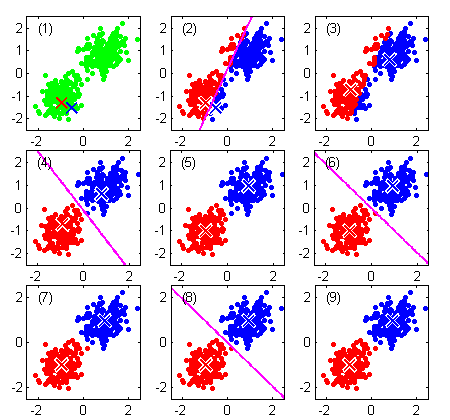图1 Kmeans聚类过程图示

2. 图像分割应用

彩色图像中的每一个像素是三维空间中的一个点，三维对应红、绿、蓝三原色的强度，基于Kmeans聚类算法的图像分割以图像的像素为数据点，按照指定的簇数进行聚类，然后将每个像素点以其对应的聚类中心替代，重构该图像。如图2所示，不同的聚类簇数呈现不同的色彩特征。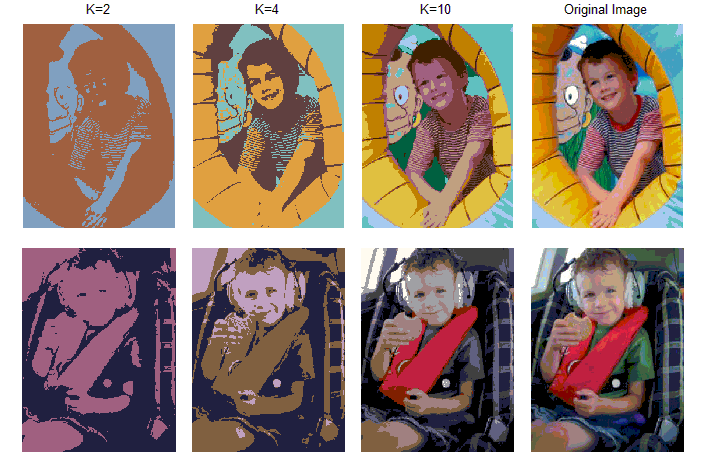图2 Kmeans用于图像分割

3. Kmeans聚类的缺点

1) 聚类簇数K没有明确的选取准则，但是在实际应用中K一般不会设置很大，可以通过枚举法，比如令K从2到10。其实很多经典方法的参数都没有明确的选取准则，如PCA的主元个数，可以通过多次实验或者采取一些小技巧来选择，一般都会达到很好的效果。
2) 从Kmeans算法框架可以看出，该算法的每一次迭代都要遍历所有样本，计算每个样本到所有聚类中心的距离，因此当样本规模非常大时，算法的时间开销是非常大的。
3) Kmeans算法是基于距离的划分方法，只适用于分布为凸形的数据集，不适合聚类非凸形状的类簇，如图3所示。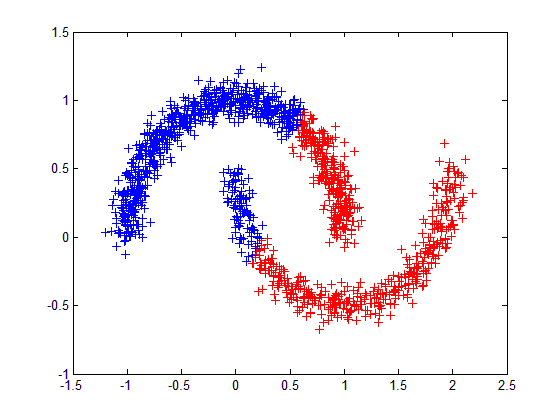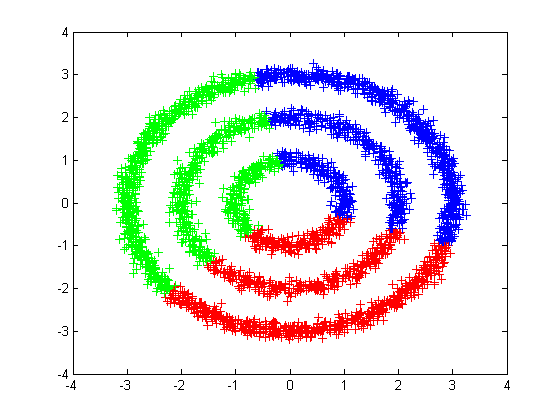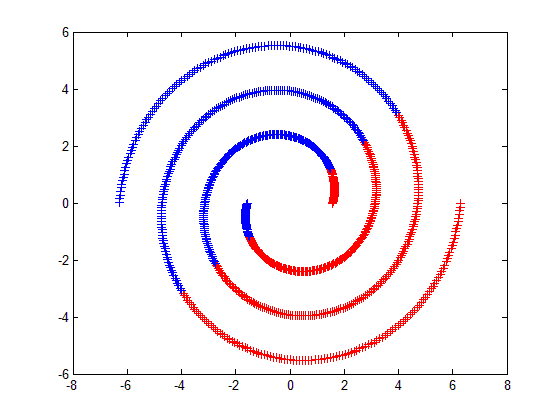图3 Kmeans聚类的缺点

展开全文jteng 2015-09-29 21:37:08
• mean shift图像分割 Reference: Mean shift: A robust approach toward feature space analysis, PAMI, 2002 mean shift,非常好的ppt，百度文库链接  Pattern Recognition and Machine Learning, ...

mean shift 图像分割

Reference:

 Mean shift: A robust approach toward feature space analysis, PAMI, 2002

 mean shift,非常好的ppt ，百度文库链接

 Pattern Recognition and Machine Learning, Bishop, 2006，Sec 2.5

 Computer Vision Algorithms and Applications, Richard Szeliski, 2010, Sec 5.3

 Kernel smoothing,MP Wand, MC Jones ,1994, Chapter 4

mean shift 图像分割 (一): 1 总体思想，2 算法步骤

mean shift 图像分割 (二): 3 算法原理，4 延伸

mean shift 图像分割 (三): 5 非参数密度估计

图像分割—mean shift(OpenCV源码注解)

写在前头的话：这篇笔记看起来公式巨多，实际上只是符号表示，没啥公式推导，不过，多了就难免有差错，欢迎指正。

Mean shitf的故事说来挺励的，早在1975年就诞生了，接着就是漫长的黑暗岁月，黑暗到几乎淡出了人们的视野，不过，命运总是善良的，95年又重新焕发生机，各种应用喷薄而出，包括目标跟踪，边缘检测，非极大值抑制等。这次就只介绍在图像分割中的应用吧，其它的我也没看。Mean shitf过程也充满正能量，描绘的是如何通过自己的努力，一步一步爬上顶峰的故事。

1 总体思想

图 1 特征空间映射：RGB图片 -> L-u特征空间

首先meanshift是一种特征空间分析方法，要利用此方法来解决特定问题，需要将该问题映射到特征空间。对于图像分割，我们可以映射到颜色特征空间，比如将RGB图片，映射到Luv特征空间，图1是L-u二维可视化的效果。

图像分割就是求每一个像素点的类标号。类标号取决于它在特征空间所属的cluster。对于每一个cluster，首先得有个类中心，它深深地吸引着一些点，就形成了一个类，即类中心对类中的点构成一个basin of attraction ，好比咱们的太阳系。如此，图像分割问题，就可以看成对每个像素点，找它的类中心问题，因为找到类中心就知道它是属于那一类啦，即类中心一样的点就是一类。

图2标准化后的概率密度可视化效果 -> 聚类分割结果

密度估计的思路需要解决两个问题，what:中心是什么？how：怎么找？mean shift认为中心是概率密度（probalility density function ）的极大值点，如图2中的红色点，原文称之为mode，我这暂且用模点吧（某篇论文是如此称呼）。对于每个点怎样找到它的类中心呢？只要沿着梯度方向一步一步慢慢爬，就总能爬到极值点，图2中黑色的线，就是爬坡的轨迹。这种迭代搜索的策略在最优化中称之为 multiple restart gradient descent。不过，一般的gradient descent并不能保证收敛到局部极值，但mean shift 可以做到，因为它的步长是自适应调整的，越靠近极值点步长越小。

也就是说meanshift的核心就两点，密度估计(Density Estimation) 和mode 搜索。对于图像数据，其分布无固定模式可循，所以密度估计必须用非参数估计，选用的是具有平滑效果的核密度估计（Kernel density estimation，KDE）。

2 算法步骤

截取这一块可视化

（a）灰度图可视化à（b）mean shift模点路径à（c）滤波后效果à（d）分割结果

分三步走：模点搜索/图像平滑、模点聚类/合并相似区域、兼并小区域（可选）。模点搜索是为了找到每个数据点的到类中心，以中心的颜色代替自己的颜色，从而平滑图像。但模点搜索得到的模点太多，并且很多模点挨得很近，若果将每个模点都作为一类的话，类别太多，容易产生过分割，即分割太细，所以要合并掉一些模点，也就是合并相似区域。模点聚类后所得到的分割区域中，有些区域所包含的像素点太少，这些小区域也不是我们想要的，需要再次合并。

2.1 模点搜索/图像平滑

建议先看中的演示（P4-12）

图像中的点包括两类信息：坐标空间（spatial，，），颜色空间（range ，，）。这些就构成了特征空间。

模点搜索（OpenCV）：某一个点，它在联合特征空间中迭代搜索它的mode/模点；

图像平滑: 将模点的颜色值赋给它自己，即.对应原文中的图像平滑，实质上是通过模点搜索，达到图像平滑的效果, 所以我合并为以一步。

设点依次爬过的脚印为：

出发时，它所收敛到的模点为，c代表convergence。

第一步：如果迭代次数超过最大值（默认最多爬5次），结束搜索跳到第四步，否则，在坐标空间，筛选靠近的数据点进入下一步计算。

OpenCV是以的坐标 为中心，边长为的方形区域内的数据点。

其实，本应用为中心，为半径的圆形区域，那样效果更好，但是循环计算时并不方便，所以用方形区域近似。

第二步：使用第一步幸存下来的点计算重心，并向重心移动。

写得有点复杂了，下面解释下。是某种核函数，比如高斯分布， 是颜色空间的核平滑尺度。OpenCV使用的是最简单的均匀分布：

二维可视化效果

是一个以（第步位置的颜色值）为球心，半径为的球体，球体内部值为1，球体外部值为0。对于经过上一步筛选后幸存的数据点，如果其颜色值满足，也就是颜色值落也在球内，那么求重心时，就要算上，否则落在球外，算重心时，就不带上它。实际上，上一步是依据坐标空间距离筛选数据点，是依据颜色距离进一步筛选数据点，上一步的筛子是矩形，这一步是球体。

简而言之，设满足的点依次为，那么重心计算公式可以进一步化简为：

是不是很简单呢，初中知识吧。

注意：上文中的两个参数，是Mean shift最核心的两个参数（还有一个可选的M），具有直观的意义，分别代表坐标空间和颜色空间的核函数带宽。

第三步：判断是否到模点了，到了就停止。

如果，移动后颜色或者位置变化很小，则结束搜索，跳到第四步，否则重返第一步，从继续爬。

OpenCV停止搜索的条件：

（1）坐标距离不变

（2）颜色变化值很小。

满足一条就可以功成身退，否则继续努力。

第四步：将模点的颜色赋给出发点/，即。

注意：原文这一步，不仅将模点的颜色值赋给，顺带把坐标值也赋给了，也就是说。

2.2 合并相似区域/模点聚类

合并上一步平滑后的图像。OpenCV采用flood fill函数实现，原理很简单，看下wiki的动画就知道了，模拟洪水浸满峡谷的效果。基本上就是区域生长，从某一点出发，如果和它附近的点（4/8邻域）的颜色值相似就合并，同时再从新合并的点出发继续合并下去，直到碰到不相似的点或者该点已经属于另一类了，此时，就退回来，直到退无可退（所有的4/8邻域搜索空间都已经搜索完毕）。

虽然很简单，但是不同的方法还是有很多需要注意的细节问题。这里假设滤波后的图像用表示。

滤波后的两个像素点和，是否合并，可以使用颜色相似度和空间位置相似性判定。

OpenCV只考虑颜色相似性，而忽略模点的坐标是否相似。而原算法综合了二者的信息。如果像素点，满足或者, 则这两个像素点就合并。不过OpenCV也是有考虑坐标位置的，它是只考虑原空间的4/8邻域，而原文是考虑特征空间模点的 ，相当于说OpenCV的（原空间）。

此外，floodfill有一个特点，它不能越过已经被分类的区域，再加上没有第三步，使得OpenCV的结果，真的是惨不忍睹。原文的合并算法，具体怎么合并的还得看源代码。不过，应该不是用flood fill。

《Computer Vision A Modern Approach》中是使用类平均距离判定是否合并。比如，，能否合并成，取决于类平均距离：

这样做我觉得效果会更好，因为它不是单独依据边界上的两个点来判定是否合并，它是依据两个区域内部所有的点的信息综合判断。所以，它能合并两个区域，而原算法和OpenCV只能是两个点合并成一个区域，该区域又不断地合并点，一旦一个区域已经完成生长，那么它就不会和别的区域合并了。可以反证。假设先形成,区域生长的时候把给合并了，那么必定有两个点满足相似关系，连接了二者，假设这两个点为，相似，那么生长的时候就肯定已经把点合并进来了，接着把所拥有的区域全盘接收，根本不会让区域自成一类。

当然考虑Outlier，使用中值更好。

假设合并之后得到m类。对于原文的算法，每个像素点的标号就是其模点所属的模点集合的类标号，比如。不过，OpenCV是所属集合的类标号。

不过，从原文结果来看，得到的结果并不是类标号，因为类标号一般都是序号，比如1，2，……，然后显示分割结果的时候，就给每一类随机分配一种独有的颜色。但原文的分割结果貌似是这一类的总体颜色值，我猜测原算法可能是用（加权）求平均的方式得到类的颜色值，然后属于这一类的像素点就用这个颜色代替。

注意：这一步实现的是合并相似区域，但本质上还是而是合并模点，或者说模点聚类，因为每个像素点的值，就是它所属模点的颜色值/模点的联合信息。
————————————————
版权声明：本文为CSDN博主「TTransposition」的原创文章，遵循 CC 4.0 BY-SA 版权协议，转载请附上原文出处链接及本声明。
原文链接：https://blog.csdn.net/ttransposition/article/details/38514127

展开全文a417197457 2020-01-09 13:04:14
• 本发明是一种基于谱聚类图像分割方法与系统，涉及聚类、机器学习和人工智能领域。特别涉及通过相关性将已经学习到的知识运用到图像分割中，并在此基础上构造性地改造谱聚类方法，从而达到快速精确地分割彩色图像的...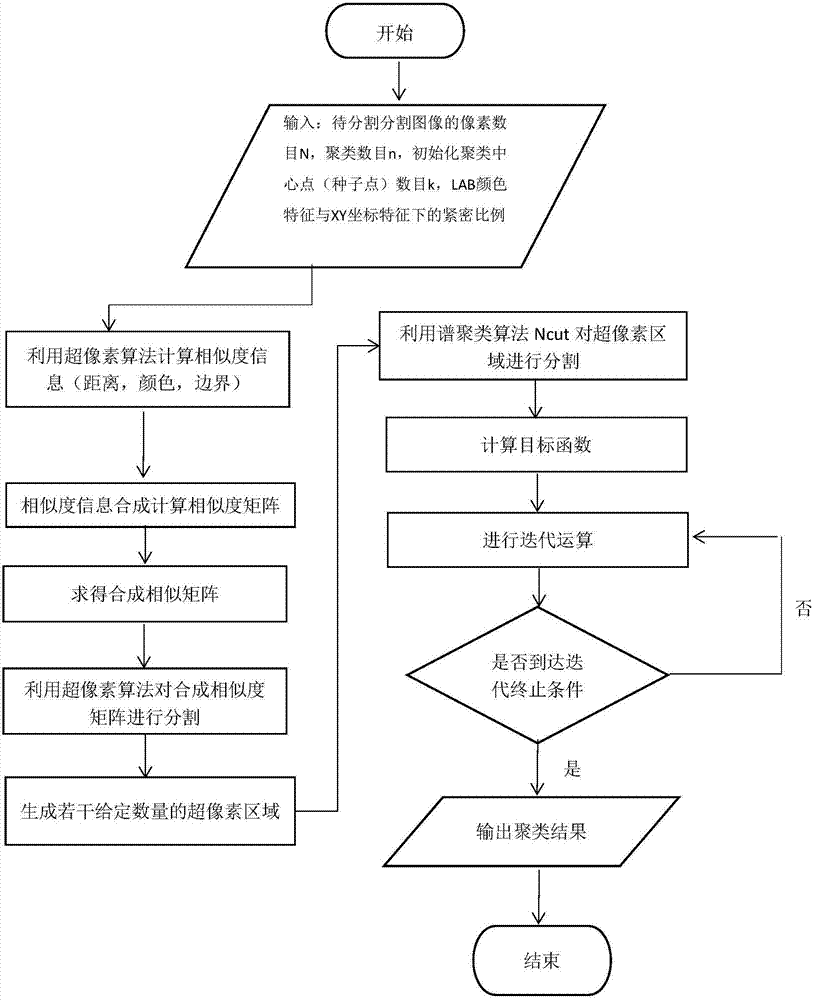本发明是一种基于谱聚类的图像分割方法与系统，涉及聚类、机器学习和人工智能领域。特别涉及通过相关性将已经学习到的知识运用到图像分割中，并在此基础上构造性地改造谱聚类方法，从而达到快速精确地分割彩色图像的目的。

背景技术：

聚类是一种无监督学习方法，主要处理目标为无标记的数据。聚类的目的是按照数据点之间的内在联系，将其划分成若干类别，使得同一类别内数据点的相似性较大，而不同类别之间数据点的相似性较小。传统的聚类方法，如k-means方法、FCM方法和EM方法等，它们虽然理论思想简单易于实现，但却缺乏处理复杂数据结构的能力，当待处理样本空间为非凸时，方法往往容易陷入局部最优。谱聚类方法由于在实际应用领域中良好的表现，以及理论简单和易于实现等特点，引起了学术界越来越多的关注。

谱聚类的理论思想建立在谱图理论的基础之上，它是数据挖掘的一种有效方法，谱聚类方法通过把数据聚类的求解问题转化成图的划分问题进行求解，以此发现不同数据之间的内在联系，谱聚类方法尤其适用于数据集非凸的情况。目前，国内外应用较多谱聚类方法在对图像进行分割时都必须建立在图像的原始像素点上。这种分割基础决定了谱聚类方法在实际应用中的处理时间过于漫长，计方法复杂度过大。可能不适用于对图像的实时处理任务，而且灵活性和可扩展性非常低。

技术实现要素：

为了解决上述问题，本发明一种基于谱聚类的图像分割方法与系统，提出基于传统谱聚类，也就是基于目前根据原始像素点处理图像的学习方法，引入超像素方法的预处理步骤，将图像的原始像素点预分割成若干像素点聚集的区域，并根据这些区域进行聚类的方法。本发明提出的方法利用已有知识与学习目标的相关性来决定聚类的方式，从而实现缩短计算时间，优化分割效果，进而使计算复杂度得以简化的学习任务。

本发明是通过以下方案实现的：

本发明一种基于谱聚类的图像分割方法与系统，首先,本文简要介绍了图像分割的基本概念,引入了图像分割的应用背景以及在数学上的定义再侧重于无监督分割方法的介绍,主要讲述了无监督图像分割的原理与当前在无监督分割领域的一些经典方法。本文研究内容如下：

(1)简要地概述了SLIC超像素方法的基础理论，其中具体包括该方法的基本理论形式、主体思想、方法的实现步骤以及处理过程。

(2)介绍了传统Ncut方法的基础理论、方法的具体实现步骤以及过程。

(3)为了降低Ncut方法的计算复杂度，提高其处理速度并减少存储负担。

本文提出了一种基于SLIC超像素方法的图像分割方法，首先采用SLIC超像素方法对原图像进行预处理操作，在这一步骤完成后可以产生数量可控的超像素区域，而后可以通过本文提出的相关公式来构造具有特定图像特征的相似性矩阵W，利用此矩阵作为传统Ncut方法的输入数据集进行图像聚类和分析。

本发明具有以下优点及效果：

(1)极大地降低了方法原本庞大的计算量，使得方法在处理过程中所必须的相似性矩阵的阶数有所减少，精减了计算时间，减少了计算机的存储负担。

(2)具有更快的学习速度。

(3)图像的最终分割效果较为良好。

本发明具体步骤如下：

(1)在原始图像上按照等距离L选取K个初始聚类中心，其中N为原始图像的像素点个数；

(2)为了避免将边界点或者奇异点当作成是聚类中心点，在这里我们尝试将聚类中心调节到原聚类中心周围3×3像素点中的梯度值为最小值的像素点；

(3)在每个聚类中心周围2L×2L的像素点区域内进行聚类，其中具体聚类的准则设置为区域像素点与其聚类中心之间的距离以及两者的亮度特征；

(4)在方法操作得到新的聚类结果中，通过选择每类区域中的每个像素点的位置以及亮度特征信息进行整合，计算其均值。

(5)若所得到的新的聚类中心与之前所得到的差异值小于事先所制订的某个阈值，则继续第6步的操作，否则，重新回到第2步，继续进行迭代聚类计算；

(6)根据公式

来计算超像素的特征信息；

(7)依据各个超像素的特征信息，用Ncut方法来把这些超像素进行聚类得到最终的分割结果。

本发明具有以下优点及效果：

(1)极大地降低了方法原本庞大的计算量，使得方法在处理过程中所必须的相似性矩阵的阶数有所减少，精减了计算时间，减少了计算机的存储负担。

(2)具有更快的学习速度。

(3)图像的最终分割效果较为良好。

附图说明

为了对本发明进一步理解，更清楚地说明本发明实施例,下面将对实施例描述中所需要使用的附图作简单介绍。

图1为本申请实施案例提供的一种基于共享近邻的约束谱聚类方法的流程图。

具体实施方式

下面将结合本申请实施例中的附图,对本申请实施例中的技术方案进行清楚、完整地描述。显然，所描述的实施案例仅仅是本申请一部分实施例。基于本申请中的实施例，本领域普通技术人员在没有付出创造性劳动前提下所获得的所有其它实施例，都属于本申请保护的范围。

实施例1

如图1所示，本实施案例包括以下步骤：

输入：原始图像，待分割图像的像素数目N，初始化聚类中心点(种子点)数目k，LAB颜色特征与XY坐标特征下的紧密比例m_compactness，聚类数目n。

输出：包含最终聚类信息的特征矩阵U

步骤1，利用超像素SLIC方法进行初始聚类。

步骤1.1：计算相似度量的设定和距离的测量。在本文中距离的测量具体可分为颜色距离以及空间距离。根据公式

对图像分割中的直线边界进行了测量，并根据公式

对图像中的位置信息和颜色信息进行了测量。

步骤1.2：对特征信息进行整合，其计算公式如下：

其中，α是经验权重，通过以上计算，可以得出每一对数据点的相似度度量。

步骤2利用谱聚类方法对生成的相似度矩阵进行分割。

计算度矩阵，其计算公式如下：

步骤2.1：计算度矩阵，其计算公式如下:

步骤2.2:构造目标函数，其计算公式如下：

subject to：X∈{0，1}N×K,X1K＝1N

步骤3：返回最终聚类结果。

最终，整个训练过程以输出阶段结束。

展开全文weixin_39765697 2021-04-23 23:53:34
• 基于聚类的图像分割-Python 计算机视觉 人工智能 opencv python 机器学习

qq_42722197 2021-11-12 01:16:14
•4星
2KB young352 2013-11-03 11:12:52
• 基于聚类的图像分割（Python） 聚类 计算机视觉 人工智能 深度学习 机器学习

dQCFKyQDXYm3F8rB0 2021-11-17 00:02:07
• 【图像分割】基于谱聚类算法实现图像分割matlab源码 matlab1

m0_60703264 2021-08-25 12:52:23
• 10KB sinat_30627027 2018-11-08 21:14:50
• ahuang1900 2013-05-31 13:02:12
• 基于聚类算法的图像分割技术 1024程序员节 聚类 算法 机器学习 图像处理

weixin_42741178 2021-10-24 13:32:51
• 【图像分割】直觉模糊C均值聚类的图像分割IFCM 聚类 算法 python 机器学习 深度学习

m0_60703264 2021-09-06 15:16:54
• 使用K-means聚类方法分割图像 C++ opencv 聚类分割 图像聚类 kmeans

Lemon_jay 2019-04-22 21:18:15
• 图像分割：K均值聚类分割（Kmeans）--python实现 Kmeans K均值 聚类 图像分割

weixin_42054926 2019-11-25 09:30:37
• 基于K均值聚类算法的图像分割（Matlab） 聚类算法 图像处理

weixin_44507817 2021-11-25 22:22:01
• 331KB weixin_38617413 2020-05-31 18:46:04
• [Python图像处理] 十九.图像分割之基于K-Means聚类的区域分割 Python图像处理 图像分割 K-Means 聚类分割 图像识别

Eastmount 2019-04-11 19:48:57
• 直觉模糊C均值聚类与图像阈值分割 模式识别 模糊聚类 阈值分割 c 图像处理

liyuefeilong 2015-02-14 11:19:16
• 【图像分割】基于直觉模糊C均值聚类实现图像分割IFCMmatlab代码 matlab 聚类 c语言

m0_60703264 2021-11-07 17:46:05
• 【MATLAB图像处理实用案例详解（7）】—— 基于K-means聚类算法的图像区域分割 聚类 算法 图像处理 matlab

didi_ya 2021-04-01 15:50:58
• 【CV】基于聚类的图像分割-Python 聚类 人工智能 机器学习 计算机视觉 深度学习

fengdu78 2021-11-16 00:56:39
• weixin_44323779 2021-04-28 23:47:25
• weixin_31748605 2021-04-27 01:37:32
• 【图像分割】基于模糊聚类算法FCM实现图像分割matlab代码 算法 matlab 聚类

qq_59747472 2021-12-12 16:44:58
• 模糊C均值聚类算法---图像分割（python） python 聚类 算法

qq_42250840 2020-06-08 21:08:56...